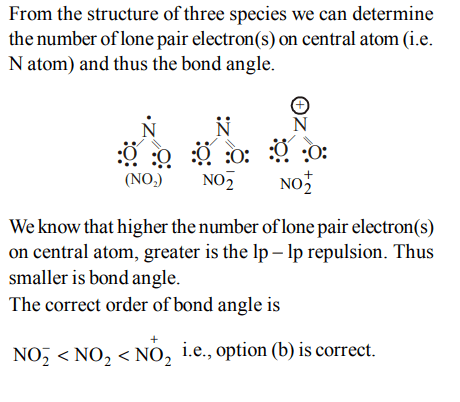## Chemical Bonding and Molecular Structure Questions and Answers Part-9

1. The number of possible resonance structure for $CO_3^{2-}$
a) 2
b) 3
c) 6
d) 9

Explanation:2. An ether is more volatile than an alcohol having the same molecular formula. This is due to
a) dipolar character of ethers
b) alcohols having resonance structures
c) inter-molecular hydrogen bonding in ethers
d) inter-molecular hydrogen bonding in alcohols

Explanation: In alcohol intermolecular H-bonding is possible whereas in ether it is not possible.

3. The reason for double helical structure of DNA is operation of
a) van der Waal’s forces
b) dipole-dipole interaction
c) hydrogen bonding
d) electrostatic attraction

Explanation: Double helical structure of DNA is stabilised by H - bonding.

4. Among the following, the molecule with the highest dipole moment is
a) $CH_{3}CI$
b) $CH_{2}CI_{2}$
c) $CHCI_{3}$
d) $CCI_{4}$

Explanation: Dipole moment follows the order CH3Cl > CH2Cl2 > CHCl3 > CCl4

5. In which of the following pairs, the two species are isostructure?
a) $SO_3^{2-} and NO_3^-$
b) $BF_{3} and NF_{3}$
c) $BrO_3^- and XeO_{3}$
d) $SF_{4} and xeF_{4}$

Explanation: Both $BrO_3^- and XeO_{3}$   have sp3 hybridization but due to presence of one lp of electrons they have trigonal pyramidal geometry.

6. The correct order of increasing bond angles in the following triatomic species is :
a) $NO_2^-< NO_2^+< NO_{2}$
b) $NO^{-}_{2}<NO_{2}<NO_2^+$
c) $NO^{+}_{2}<NO_{2}<NO_2^-$
d) $NO^{+}_{2}<NO_2^-<NO_{2}$

Explanation:7. Four diatomic species are listed below in different sequences. Which of these presents the correct order of their increasing bond order ?
a) $O_2^{-} < NO < C_2^{2-} < He_2^+$
b) $NO < C_2^{2-} < O_2^{-} < He_2^+$
c) $C_2^{2-} < He_2^+ < NO < O_2^{-}$
d) $He_2^+ < O_2^{-} < NO < C_2^{2-}$

Explanation:8. What is the dominant intermolecular force or bond that must be overcome in converting liquid CH3OH to a gas?
a) Dipole-dipole interactions
b) Covalent bonds
c) London dispersion forces
d) Hydrogen bonding

Explanation: Due to intermolecular hydrogen bonding in methanol, it exist as assosiated molecule

9. In which of the following molecules / ions $BF_{3},NO_2^-,NH_2^- and H_{2}O$    , the central atom is $sp^{2}$  hybridized ?
a) $NH_2^- and H_{2}O$
b) $NO_2^- and H_{2}O$
c) $BF_{3} and NO_2^-$
d) $NO_2^- and NH_2^-$

Explanation: On determining hybridisation from H = 1/2 (V + M – C + A). The hybridisation of $BF_{3},NO_2^-,NH_2^- and H_{2}O$      are sp2 , sp2 , sp3 and sp3 respectively
a) $N_2^{2-} < N_2^- < N_{2}$
b) $N_{2} < N_2^{2-} < N_2^-$
c) $N_2^- < N_2^{2-} < N_{2}$
d) $N_2^- < N_{2} < N_2^{2-}$